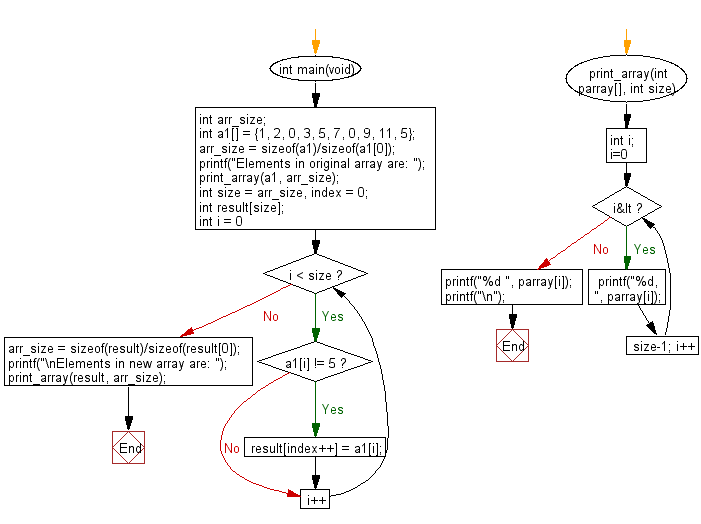﻿ C Program: Replace all 5 with 0, move all 0 to the right# C Exercises: Create a new array after replacing all the values 5 with 0 shifting all zeros to right direction

## C-programming basic algorithm: Exercise-72 with Solution

Write a C program to create a new array after replacing all the values 5 with 0 shifting all zeros to right direction.

C Code:

``````#include <stdio.h>
#include <stdlib.h>
int main(void){
int arr_size;
int a1[] = {1, 2, 0, 3, 5, 7, 0, 9, 11, 5};
arr_size = sizeof(a1)/sizeof(a1);
printf("Elements in original array are: ");
print_array(a1, arr_size);
int size = arr_size, index = 0;
int result[size];
for (int i = 0; i < size; i++)
{
if (a1[i] != 5)
{
result[index++] = a1[i];
}
}
arr_size = sizeof(result)/sizeof(result);
printf("\nElements in new array are: ");
print_array(result, arr_size);
}
print_array(int parray[], int size)
{
int i;
for( i=0; i<size-1; i++)
{
printf("%d, ", parray[i]);
}
printf("%d ", parray[i]);
printf("\n");
}
``````

Sample Output:

```Elements in original array are: 1, 2, 0, 3, 5, 7, 0, 9, 11, 5

Elements in new array are: 1, 2, 0, 3, 7, 0, 9, 11, 0, 0
```

Pictorial Presentation:Flowchart:C Programming Code Editor:

What is the difficulty level of this exercise?

Test your Programming skills with w3resource's quiz.

﻿

## C Programming: Tips of the Day

Why doesn't a+++++b work?

printf("%d",a+++++b); is interpreted as (a++)++ + b according to the Maximal Munch Rule!.

++ (postfix) doesn't evaluate to an lvalue but it requires its operand to be an lvalue.

! 6.4/4 says the next preprocessing token is the longest sequence of characters that could constitute a preprocessing token"

Ref : https://bit.ly/3fdldUT